## 4.7.2 Current

By expanding the GREEN's function in terms of the basis functions the continuity equation (3.87) can be derived as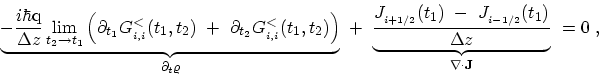(4.52)

where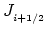represents the current passing through a point between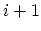and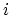. Note that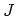has a unit of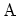rather than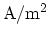due to the one-dimensional nature of CNTs. The time derivative of the GREEN's functions can be replaced by the relation (3.62)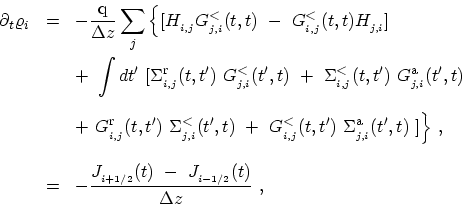(4.53)

where the term inside the integral is zero due to the condition stated in (3.90).

The next step is separatingfrom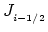by decomposing equation (4.52). CAROLI proposed the following ansatz in . The currentis the difference between the flow of particles from left to right and from right to left. This leads to the following expression for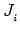(4.54)

It is straightforward to show that (4.54) along with an expression for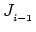satisfies (4.53).

Under steady-state condition one can transform the time difference coordinate to energy to obtain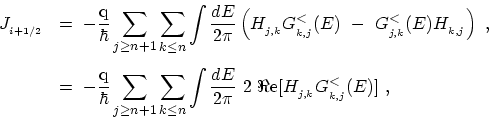(4.55)

Based on the nearest neighbor tight-binding method in mode-space (see Section 4.4) equation (4.55) can be simplified to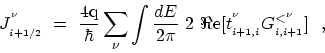(4.56)

where the summation runs over all the subbands contributing to transport. The factor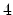in (4.56) is due to double spin and double subband degeneracy. M. Pourfath: Numerical Study of Quantum Transport in Carbon Nanotube-Based Transistors